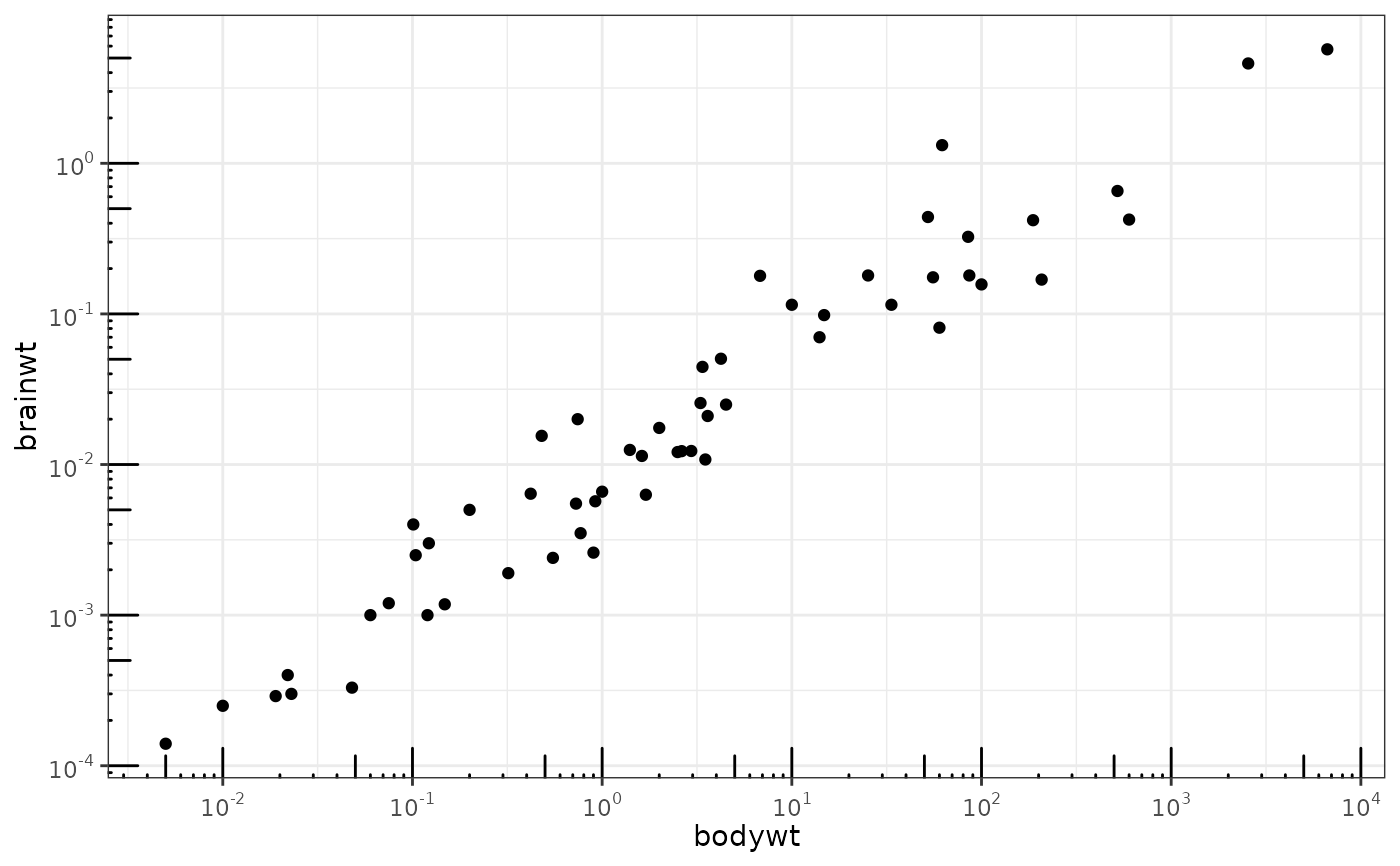This annotation adds log tick marks with diminishing spacing. These tick marks probably make sense only for base 10.

## Usage

annotation_logticks(
base = 10,
sides = "bl",
outside = FALSE,
scaled = TRUE,
short = unit(0.1, "cm"),
mid = unit(0.2, "cm"),
long = unit(0.3, "cm"),
colour = "black",
size = 0.5,
linetype = 1,
alpha = 1,
color = NULL,
...
)

## Arguments

base

the base of the log (default 10)

sides

a string that controls which sides of the plot the log ticks appear on. It can be set to a string containing any of "trbl", for top, right, bottom, and left.

outside

logical that controls whether to move the log ticks outside of the plot area. Default is off (FALSE). You will also need to use coord_cartesian(clip = "off"). See examples.

scaled

is the data already log-scaled? This should be TRUE (default) when the data is already transformed with log10() or when using scale_y_log10(). It should be FALSE when using coord_trans(y = "log10").

short

a grid::unit() object specifying the length of the short tick marks

mid

a grid::unit() object specifying the length of the middle tick marks. In base 10, these are the "5" ticks.

long

a grid::unit() object specifying the length of the long tick marks. In base 10, these are the "1" (or "10") ticks.

colour

Colour of the tick marks.

size

Thickness of tick marks, in mm.

linetype

Linetype of tick marks (solid, dashed, etc.)

alpha

The transparency of the tick marks.

color

An alias for colour.

...

Other parameters passed on to the layer

scale_y_continuous(), scale_y_log10() for log scale transformations.

coord_trans() for log coordinate transformations.

## Examples

# Make a log-log plot (without log ticks)
a <- ggplot(msleep, aes(bodywt, brainwt)) +
geom_point(na.rm = TRUE) +
scale_x_log10(
breaks = scales::trans_breaks("log10", function(x) 10^x),
labels = scales::trans_format("log10", scales::math_format(10^.x))
) +
scale_y_log10(
breaks = scales::trans_breaks("log10", function(x) 10^x),
labels = scales::trans_format("log10", scales::math_format(10^.x))
) +
theme_bw()

a + annotation_logticks()                # Default: log ticks on bottom and left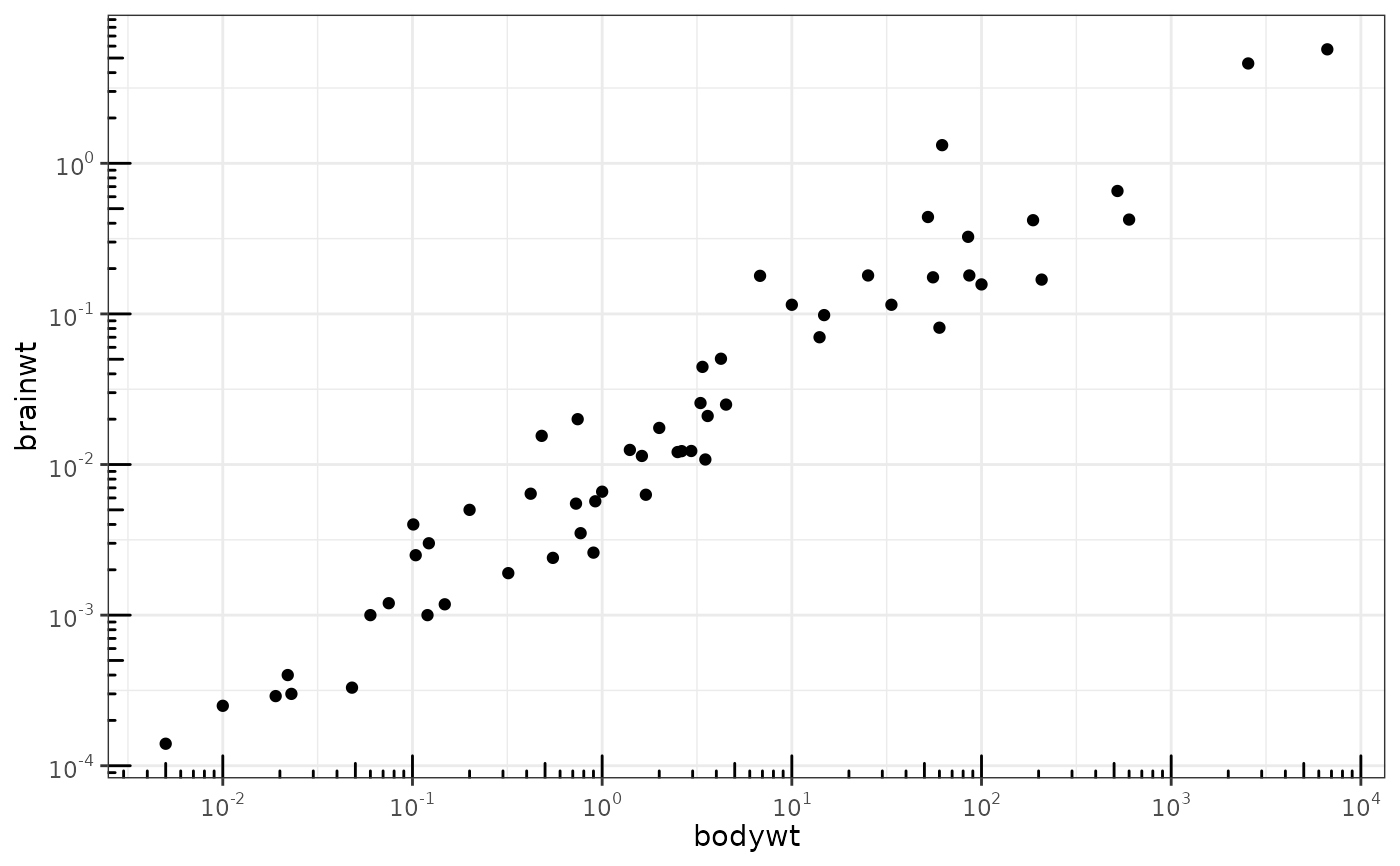a + annotation_logticks(sides = "lr")    # Log ticks for y, on left and right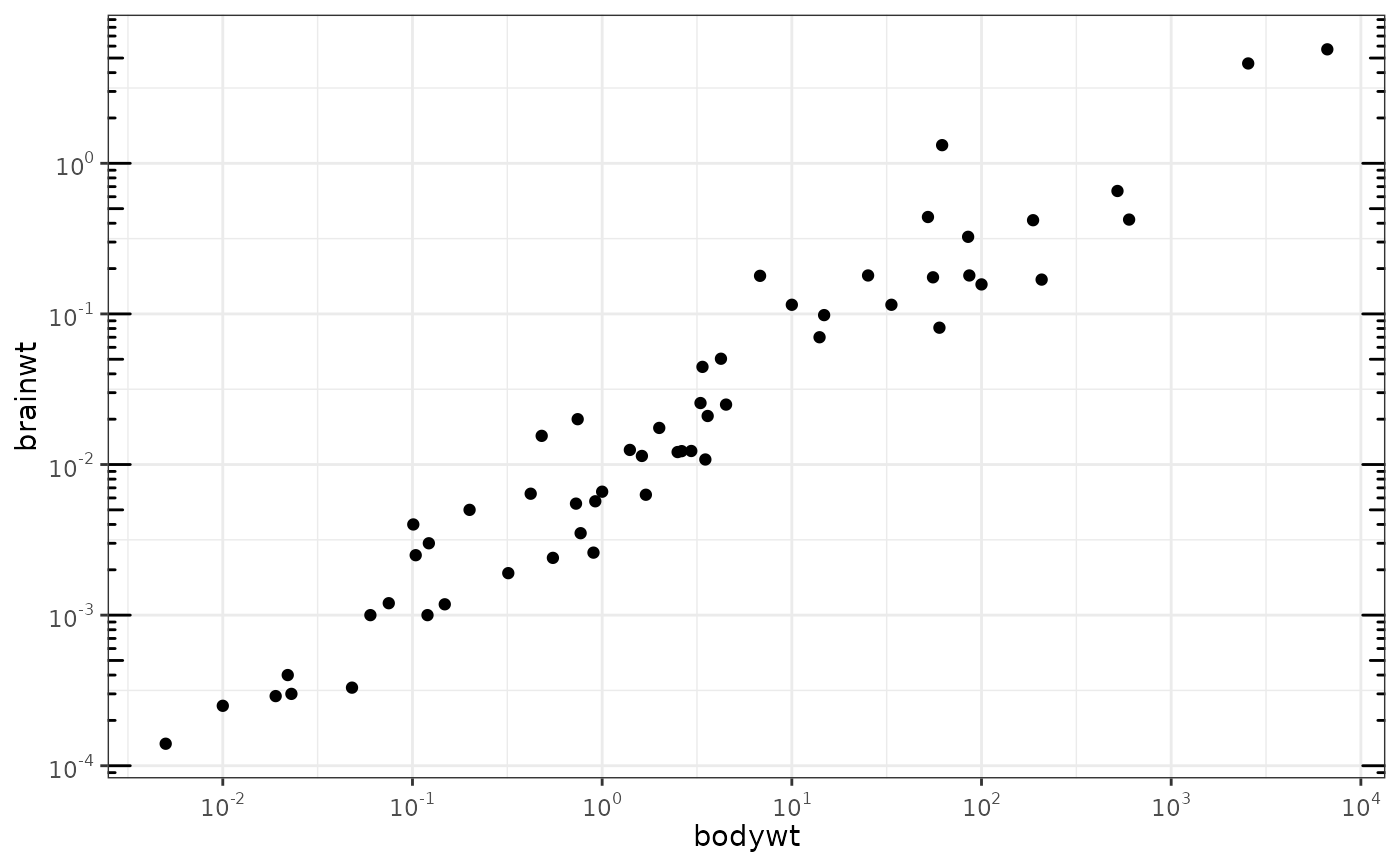a + annotation_logticks(sides = "trbl")  # All four sides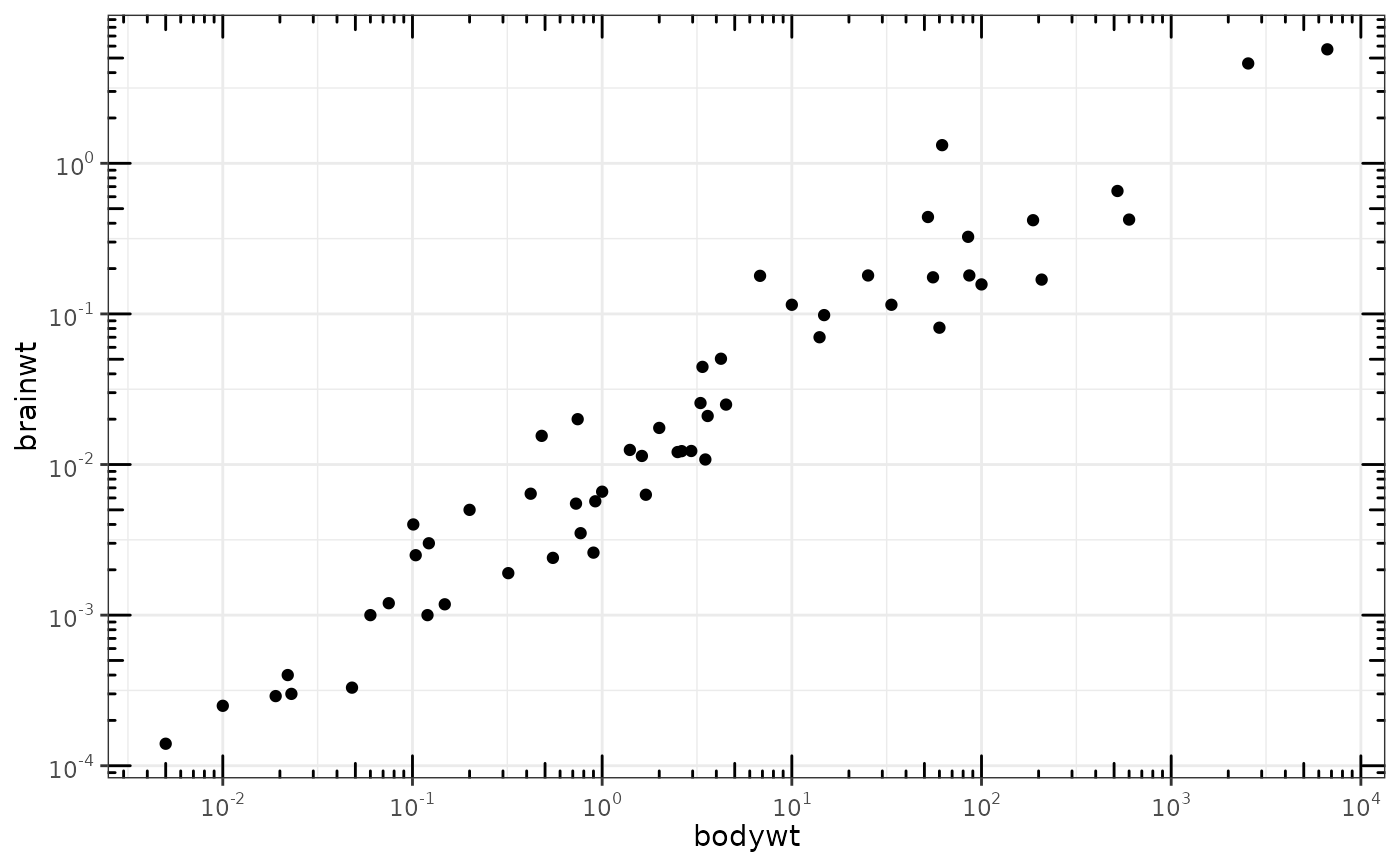a + annotation_logticks(sides = "lr", outside = TRUE) +
coord_cartesian(clip = "off")  # Ticks outside plot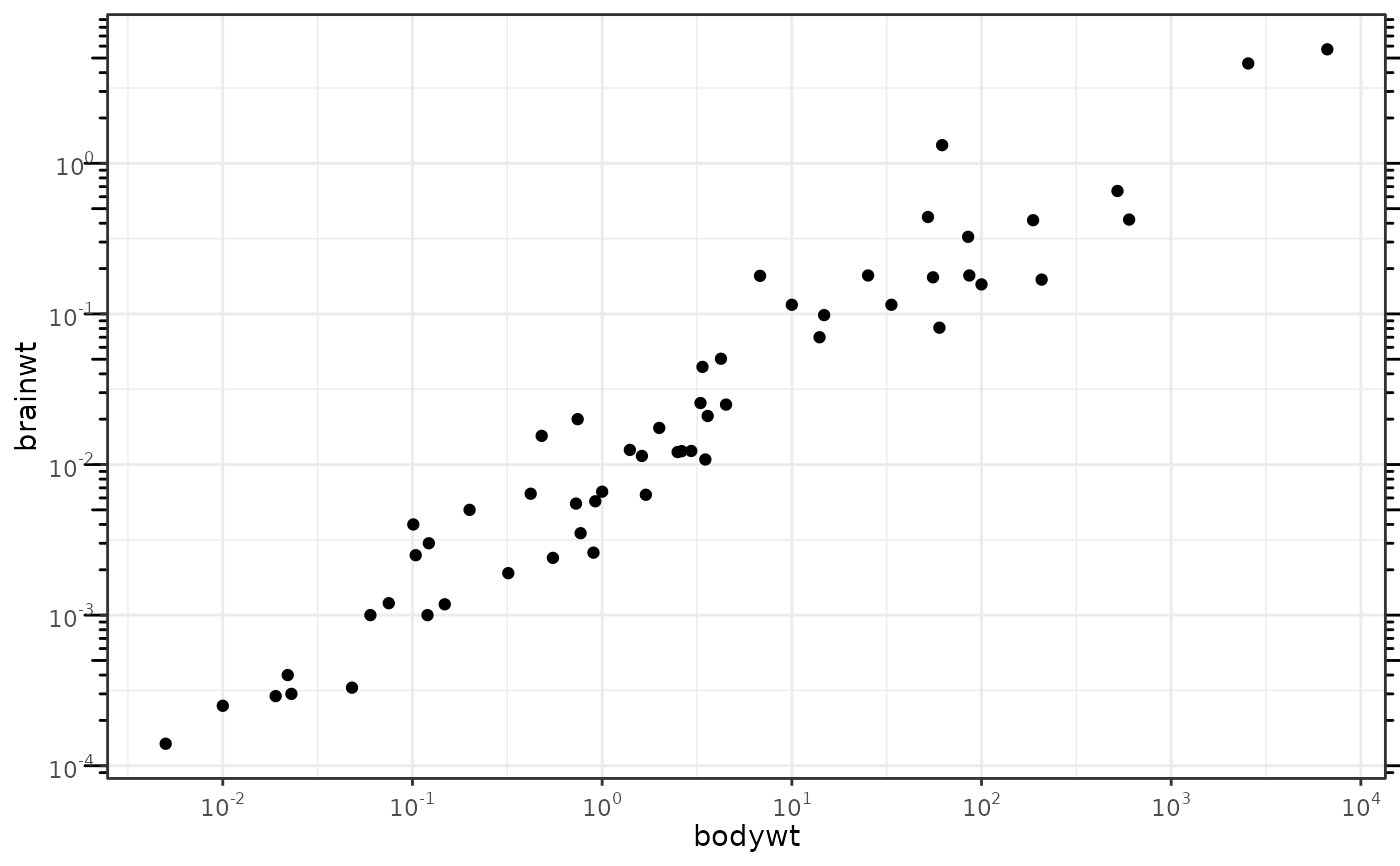# Hide the minor grid lines because they don't align with the ticks
a + annotation_logticks(sides = "trbl") + theme(panel.grid.minor = element_blank())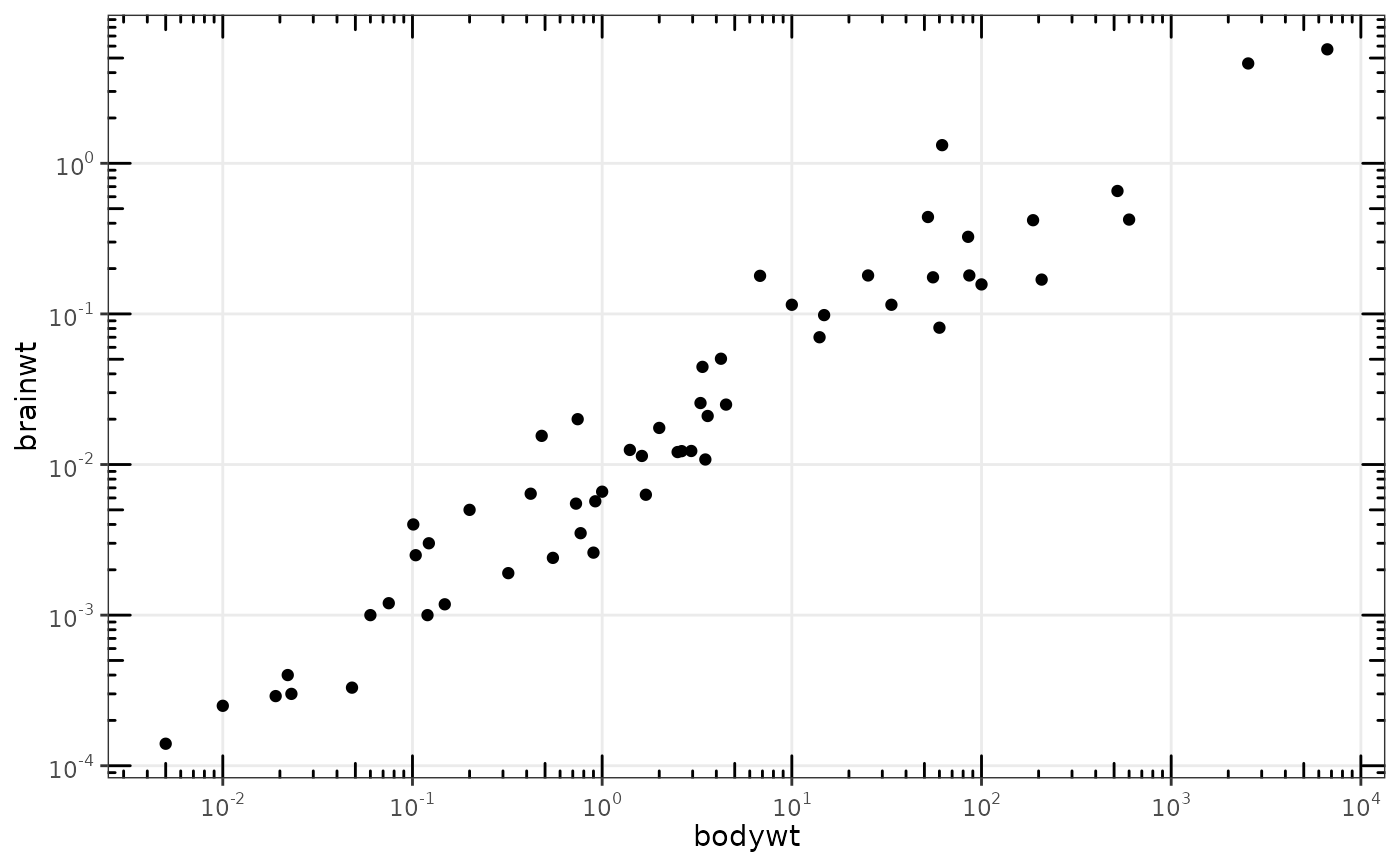# Another way to get the same results as 'a' above: log-transform the data before
# plotting it. Also hide the minor grid lines.
b <- ggplot(msleep, aes(log10(bodywt), log10(brainwt))) +
geom_point(na.rm = TRUE) +
scale_x_continuous(name = "body", labels = scales::math_format(10^.x)) +
scale_y_continuous(name = "brain", labels = scales::math_format(10^.x)) +
theme_bw() + theme(panel.grid.minor = element_blank())

b + annotation_logticks()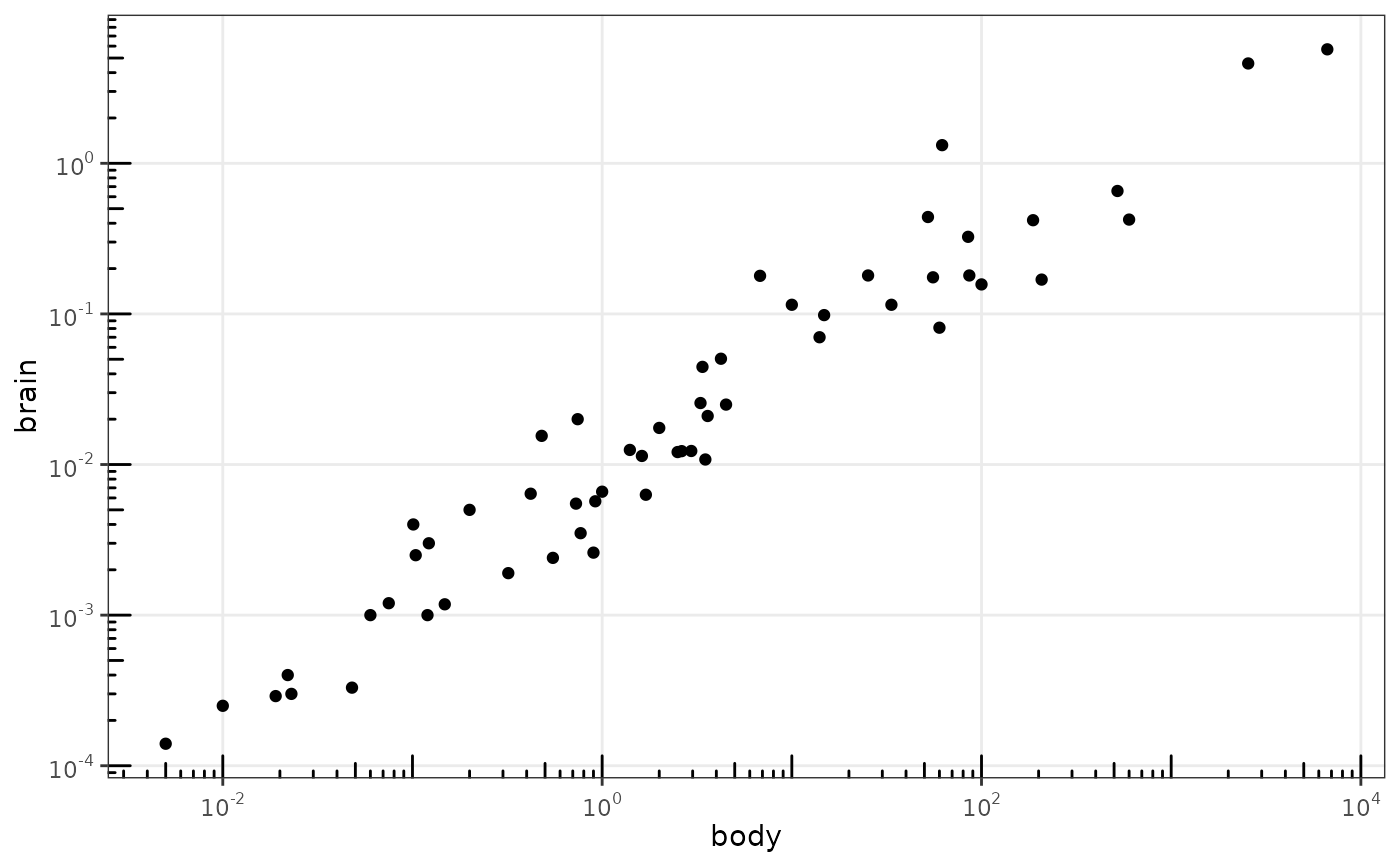# Using a coordinate transform requires scaled = FALSE
t <- ggplot(msleep, aes(bodywt, brainwt)) +
geom_point() +
coord_trans(x = "log10", y = "log10") +
theme_bw()
t + annotation_logticks(scaled = FALSE)
#> Warning: Removed 27 rows containing missing values (geom_point).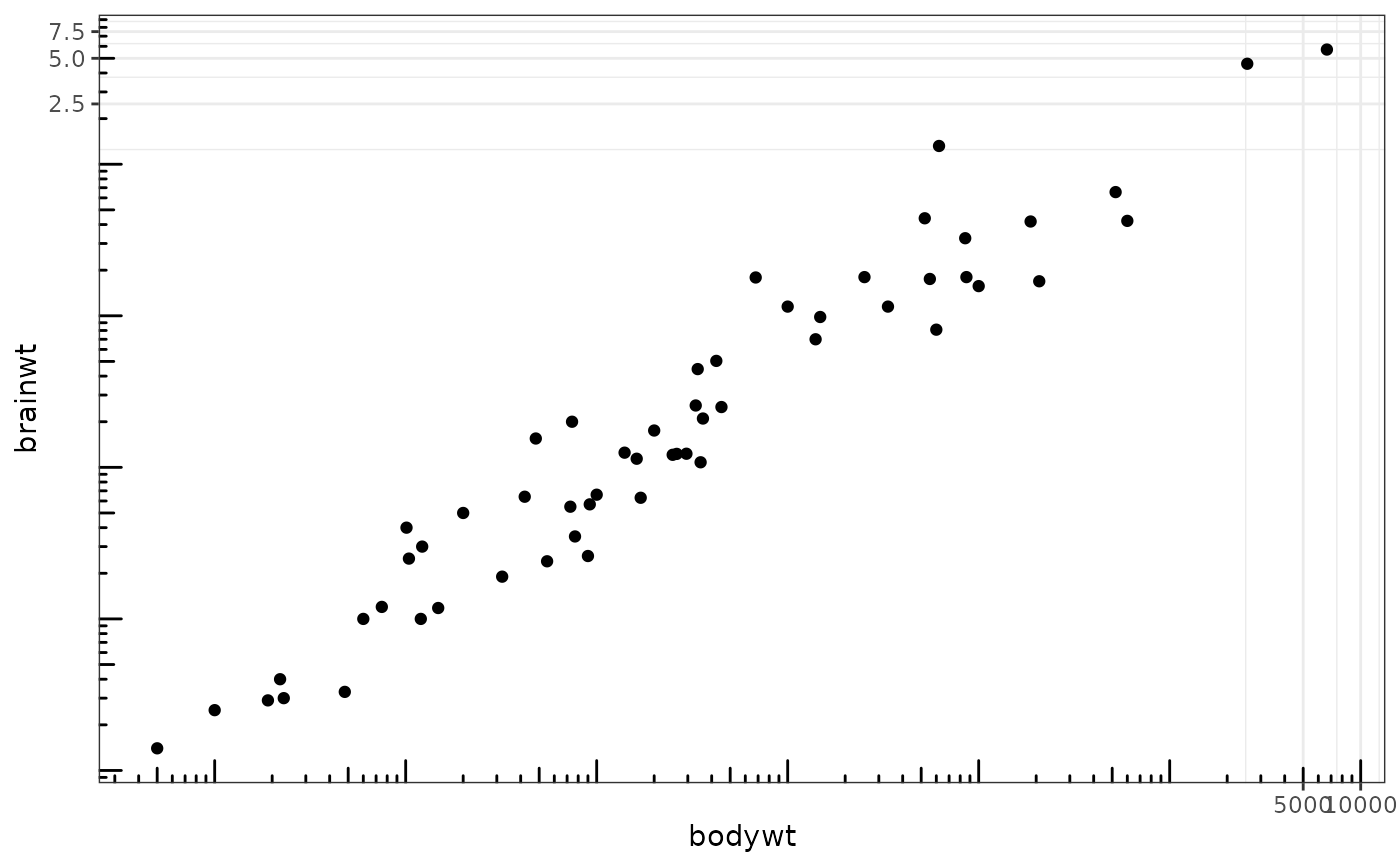# Change the length of the ticks
a + annotation_logticks(
short = unit(.5,"mm"),
mid = unit(3,"mm"),
long = unit(4,"mm")
)